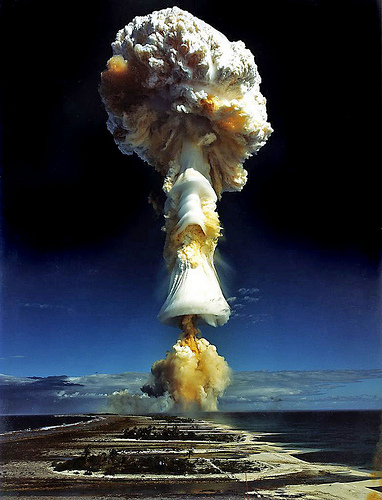# Converting Energy into Matter: What it Really MeansYou are almost certainly familiar with the popular equation: E=mc^2, but do you know what it means? If you’ve read a pop science book every now and then, or paid attention in physics and chemistry classes, you may already know that it means mass and energy are equivalent. But this doesn’t necessarily mean what you think it does, either.

First off, we need to distinguish between mass and matter. Mass is a measurable quantity related to concepts like force and momentum. The more mass an object has, the more force it takes to accelerate it at the same pace.

Matter, at this point in our understanding of physics, isn’t necessarily as easy to define as we might think it should be. Matter isn’t necessarily solid (it can be liquid, solid, gas, or plasma), or even “tangible” (neutrinos are matter, but they pass through almost everything like a ghost).

The best way to define matter with modern physics would be to say that it has the following properties:

• It is composed of fermions, elementary particles that have a “half-integer spin.” While elementary particles don’t really “spin,” this is a useful explanation because they do actually carry a quantity of “angular momentum,” and enough angular momentum will cause an object to spin.
• They are limited to speeds lower than the speed of light.
• They have mass when they are not moving (you’ll later see why we made this odd comment).
• No two fermions can occupy the exact same quantum state. For example, two electrons carrying the same amount of energy as they oscillate around an atom can’t have the same spin. All electrons must have the same quantity of spin, so they must spin in opposite directions.

Why is it so important to draw a distinction between mass and matter? Because energy cannot be physically converted into mass, but it can be physically converted into matter.

Confused yet?

The equation E=mc^2 doesn’t tell us that mass and energy can be physically transformed into one another. Instead, it says they are actually identical, and that it is meaningless to differentiate between them. For convenience, we use one system of units for mass and another for energy, but they are actually one and the same.

For example, when an atom absorbs a neutron, it will release a gamma ray (energy). The loss of this gamma ray will cause the actual mass of the atom to decrease. This phenomenon was demonstrated accurate to 0.0004% in 2005. Furthermore, if this gamma ray is absorbed by a different atom, it will cause the atom’s mass to increase.

Of course, an important thing to recognize about E=mc^2 is that c is the speed of light, a very big number. So what we would consider a small amount of mass, we would consider a large amount of energy. But, again, energy in all its forms is mass, and mass in all its forms is energy.

Some mistakenly think that mass-energy equivalence breaks the laws of conservation of mass and energy, rewriting them as a new law that explains how one can be turned into another. Instead, it explains that neither mass nor energy can be created or destroyed, but they are identical.

It’s also important to define energy itself, of course. Like mass, energy is not a mechanism or a certain type of particle, it is a measurable quantity. Energy measures the capability of a system to do “work,” which is defined as force times distance.

When you push a boulder up a hill, you are transforming energy from food into energy of motion, called kinetic energy, through work. The boulder, in turn, will transform this kinetic energy mostly into heat as it rubs against the ground. It accomplishes this by doing work on the ground, transforming its energy into the kinetic energy of billions of tiny atoms.

What we have learned is that we can also transform energy into matter. This is accomplished in particle accelerators on a daily basis. Particles are accelerated to close to the speed of light, so that they accumulate a relatively large amount of kinetic energy. The particles are then collided with one another. This collision transforms the kinetic energy into matter, which is how we were able to discover various kinds of new elementary particles.

Hopefully now you can see why we made the odd comment that particles of matter have mass when they aren’t moving, which is called “rest mass.” Since energy and mass are equivalent, and motion creates kinetic energy, motion creates mass.

By the same token, matter can be converted into energy, which is how nuclear bombs and power plants work.

The takeaways here are that matter is just one kind of energy, but energy and mass are identical.

For more on relativity, check out my post: The Theory of Relativity, For Kids.

Related: Simply Einstein: Relativity DemystifiedStudy: Debt Crisis Caused by the Educated